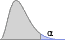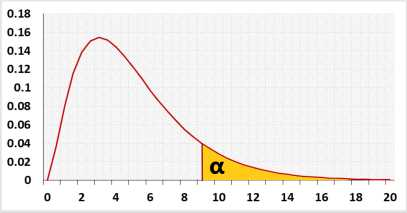# Chi-squared Statistical power calculatorStatistical power chart (real values)
Legend (right tail example)

H0:H1:## Information

Calculates the test power based on the sample size and draw the power analysis chart.
Use this calculator for one of the following tests:
Goodness of fit test calculator
Degrees of freedom - the number of categories minus one.
Sample size - the total number of observations across the categories.
Chi-Squared test For variance calculator
Degrees of freedom - the total number of observations minus one.
Sample size - the total number of observations.

### χ² Distribution## R Code

The following R code should produce the same results